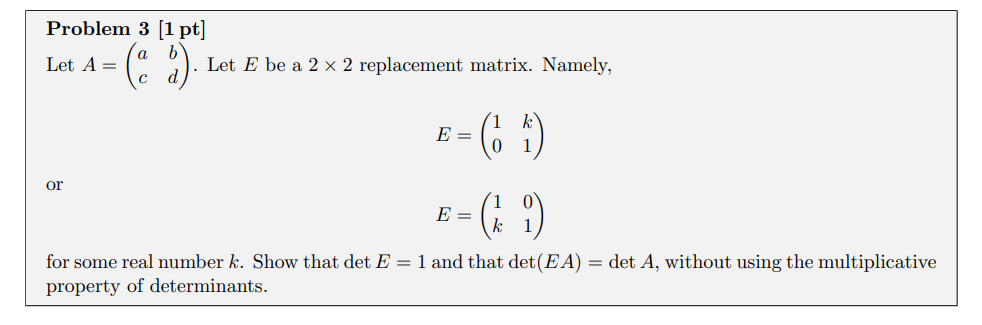Home / Expert Answers / Other Math / let-a-acbd-let-e-be-a-22-replacement-matrix-namely-e-10k1-or-e-1k01-f-pa415

# (Solved): Let A=(acbd). Let E be a 22 replacement matrix. Namely, E=(10k1) or E=(1k01) f ...Let . Let be a replacement matrix. Namely, or for some real number . Show that and that , without using the multiplicative property of determinants.

We have an Answer from Expert